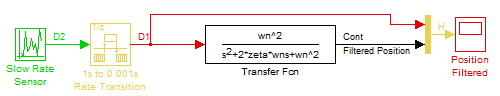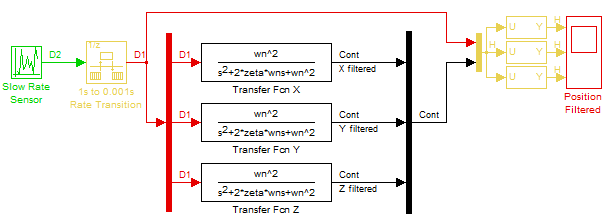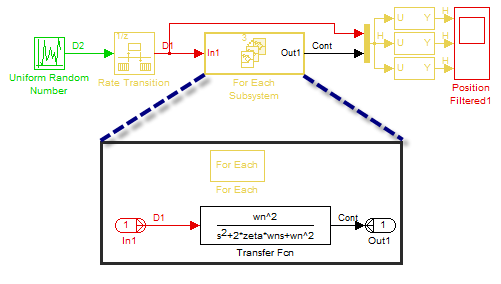# Continuous sample time inside For Each Subsystem

Back in 2010, I wrote a post explaining how to apply a scalar algorithm to a vector using the For Each subsystem. At that time, only discrete algorithms could be vectorized that way. In R2011b, the For Each subsystem now supports continuous sample times.

Let's look at a simple example.

Filtering sensors data

In multiple occasions in the past I ended up acquiring the signal of a slow rate sensor and using this signal into a fast rate controller. In order avoid feeding a discontinuous signal to my controller, I used to insert a Transfer Function block to filter the slow rate signal. A simplified version of this processing look like this:The problem is that often the sensors do not provide a scalar value, but a vector. For example, a vision system can return the three components of a Cartesian position. In that case, I had to separate the components and filter them individually:For a vector of three elements, this is not too bad. But I have seen models where hundreds of signals had to be separated and recombined. In R2011b, all you have to do is to put the Transfer Function block inside the For Each Subsystem, and it will adapt automatically to the number of elements of the input signal.In addition to making the model easier to edit, it also speeds up the model initialization and generate more efficient code, because the model contains less blocks.

Now it's you turn

For a more complex example, I recommend looking at the demo titled Modeling Objects with Identical Dynamics using For Each Subsystem.

How are you going to take advantage of the continuous sample time support inside the For Each Subsystem? Modeling a farm of wind turbines? Multiple planes flying together? An army of identical robots? Leave a comment here.

|